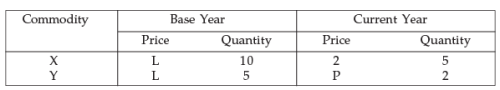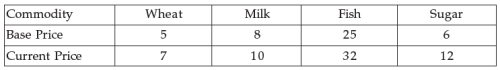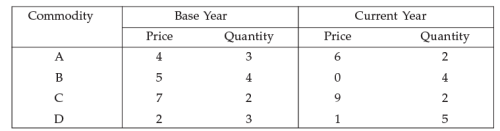# Test: Index Numbers- 2

## 40 Questions MCQ Test Quantitative Aptitude for CA CPT | Test: Index Numbers- 2

Description
This mock test of Test: Index Numbers- 2 for CA Foundation helps you for every CA Foundation entrance exam. This contains 40 Multiple Choice Questions for CA Foundation Test: Index Numbers- 2 (mcq) to study with solutions a complete question bank. The solved questions answers in this Test: Index Numbers- 2 quiz give you a good mix of easy questions and tough questions. CA Foundation students definitely take this Test: Index Numbers- 2 exercise for a better result in the exam. You can find other Test: Index Numbers- 2 extra questions, long questions & short questions for CA Foundation on EduRev as well by searching above.
QUESTION: 1

Solution:
QUESTION: 2

Solution:
QUESTION: 3

### Simple aggregate of quantities is a type of

Solution:
QUESTION: 4

We use price index numbers

Solution:
QUESTION: 5

Each of the following statements is either True or False write your choice of the answer by writing T for True

Solution:
QUESTION: 6

Each of the following statements is either True or False. Write your choice of the answer by writing F for false.

Solution:
QUESTION: 7

The best average for constructing an index numbers is

Solution:
QUESTION: 8

The time reversal test is satisfied by

Solution:
QUESTION: 9

The factor reversal test is satisfied by

Solution:
QUESTION: 10

The circular test is satisfied by

Solution:
QUESTION: 11

Fisher’s index number is based on

Solution:
QUESTION: 12

Paasche index is based on

Solution:
QUESTION: 13

Fisher’s ideal index number is

Solution:
QUESTION: 14

Price-relative is expressed in term of

Solution:
QUESTION: 15

Paasehe’s index number is expressed in terms of :

Solution:
QUESTION: 16

Time reversal Test is satisfied by following index number formula is

Solution:
QUESTION: 17

Cost of living Index number (C. L. I.) is expressed in terms of :

Solution:
QUESTION: 18

If the ratio between Laspeyre’s index number Paasche’s Index number is 28 : 27. Then the Missing figure in the following table P is :Solution:
QUESTION: 19

If the prices of all commodities in a place have increased 1.25 times in comparison to the base period, the index number of prices of that place is now

Solution:

According to the question,  Given : P1 = P0 + 1.25 P0
P1  = 2.25 P0
In index numbers, we assume base price P0 = 100
P1 = 2.25 ( 100)
P1  = 225
Price of that place = 225

QUESTION: 20

If the index number of prices at a place in 1994 is 250 with 1984 as base year, then the prices have increased on average

Solution:
QUESTION: 21

If the prices of all commodities in a place have decreased 35% over the base period prices, then the index number of prices of that place is now

Solution:
QUESTION: 22

Link relative index number is expressed for period n is

Solution:
QUESTION: 23

Fisher’s Ideal Index number is expressed in terms of :

Solution:
QUESTION: 24

Factor Reversal Test According to Fisher is

Solution:
QUESTION: 25

Marshall Edge worth Index formula after interchange of p and q is impressed in terms of :

Solution:
QUESTION: 26

If ∑ Pnqn= 249, ∑ Poqo = 150, Paasche’s Index Number = 150 and Drobiseh and Bowely’s Index number = 145. Then the Fisher’s Ideal Index Number is

Solution:
QUESTION: 27

Consumer Price index number for the year 1957 was 313 with 1940 as the base year 96 the Average Monthly wages in 1957 of the workers into factory be Rs. 160/- their real wages is

Solution:
QUESTION: 28

If ∑ Poqo = 3500, ∑ Pnqo = 3850. Then the Cost of living Index (C.L.T.) for 1950 w.r. to base 1960 is

Solution:
QUESTION: 29

From the following table by the method of relatives using Arithmetic mean the price Index number isSolution:
QUESTION: 30

Each of the following statements is either True or False with your choice of the answer by writing F for False.

Solution:
QUESTION: 31

Each of the following statements is either True or False with your choice of the answer by writing F for False.

Solution:
QUESTION: 32

Each of the following statements is either True or False with your choice of the answer by writing F for False.

Solution:
QUESTION: 33

Each of the following statements is either True or False with your choice of the answer by writing F for False.

Solution:
QUESTION: 34

In 1980,the net monthly income of the employee was Rs. 800/- p. m. The consumer price index number was 160 in 1980. It rises to 200 in 1984. If he has to be rightly compensated.The additional D. A. to be paid to the employee is

Solution:
QUESTION: 35

The simple Aggregative formula and weighted aggregative formula satisfy is

Solution:
*Multiple options can be correct
QUESTION: 36

“Fisher’s Ideal Index is the only formula which satisfies”

Solution:
QUESTION: 37

“Neither Laspeyre’s formula nor Paasche’s formula obeys” :

Solution:
QUESTION: 38

The aggregative expenditure method and family budget method always give:

Solution:
QUESTION: 39

With the base year 1960 as the base the C. L. I. In 1972 stood at 250 x was getting a monthly Salary of Rs. 500 in 1960 and Rs. 750 in 1972. In 1972 to maintain his standard of living in 1960 x have received as extra allowances is

Solution:
QUESTION: 40

From the following data base year :-Fisher’s Ideal Index is

Solution: